# The SYSLIN Procedure

### The R-Square Statistics

As explained in the section ANOVA Table for Instrumental Variables Methods, when instrumental variables are used, the regression sum of squares (RSS) and the error sum of squares (ESS) do not sum to the total corrected sum of squares. In this case, there are several ways that the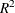statistic can be defined.

The definition ofused by the SYSLIN procedure is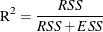This definition is consistent with the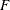test of the null hypothesis that the true coefficients of all regressors are zero. However, thismight not be a good measure of the goodness of fit of the model.

#### System Weighted R-Square and System Weighted Mean Squared Error

The system weighted, printed for the 3SLS, IT3SLS, SUR, ITSUR, and FIML methods, is computed as follows.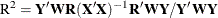In this equation, the matrixis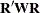and W is the projection matrix of the instruments: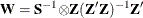The matrix Z is the instrument set, R is the regressor set, and S is the estimated cross-model covariance matrix.

The system weighted MSE, printed for the 3SLS, IT3SLS, SUR, ITSUR, and FIML methods, is computed as follows: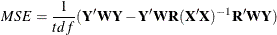In this equation, tdf is the sum of the error degrees of freedom for the equations in the system.